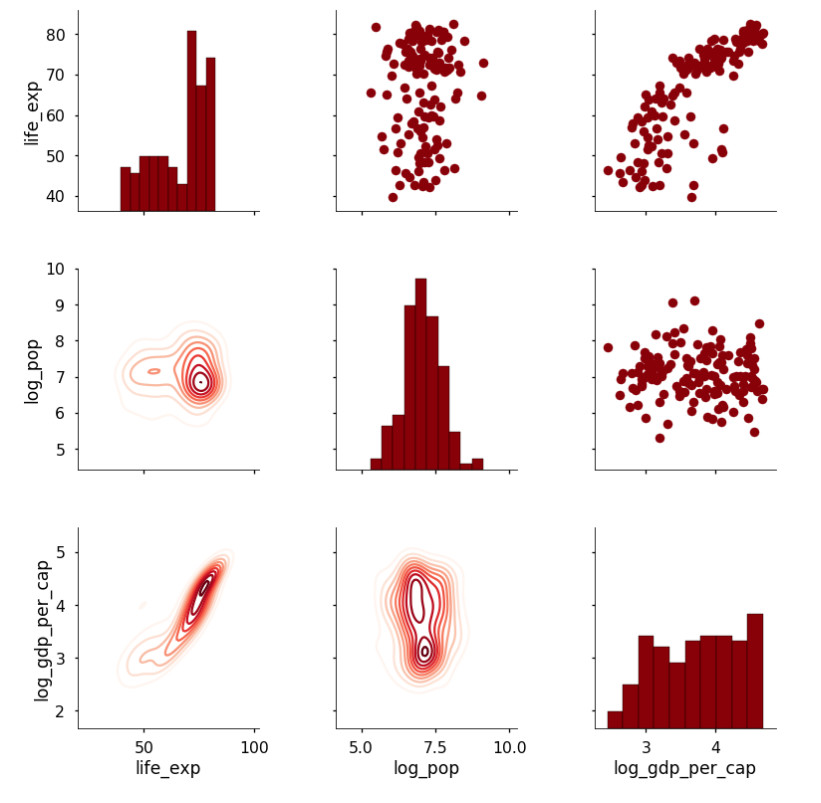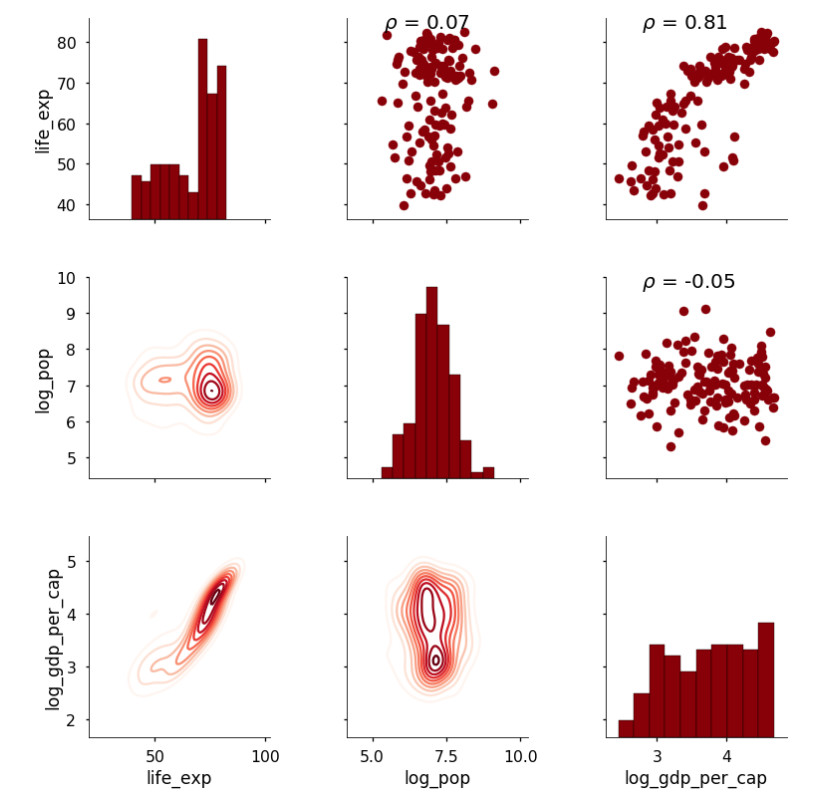# 如何利用散点图矩阵进行数据可视化

Seaborn 中的散点图矩阵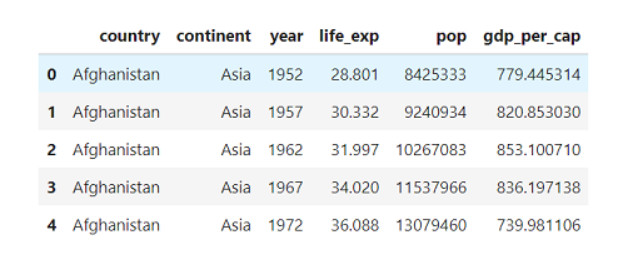seaborn 中的默认散点图矩阵仅仅画出数值列，尽管我们随后也会使用类别变量来着色。创建默认的散点图矩阵很简单：我们加载 seaborn 库，然后调用 pairplot 函数，向它传递我们的数据帧即可：

# Seaborn visualization library
import seaborn as sns
# Create the default pairplot
sns.pairplot(df)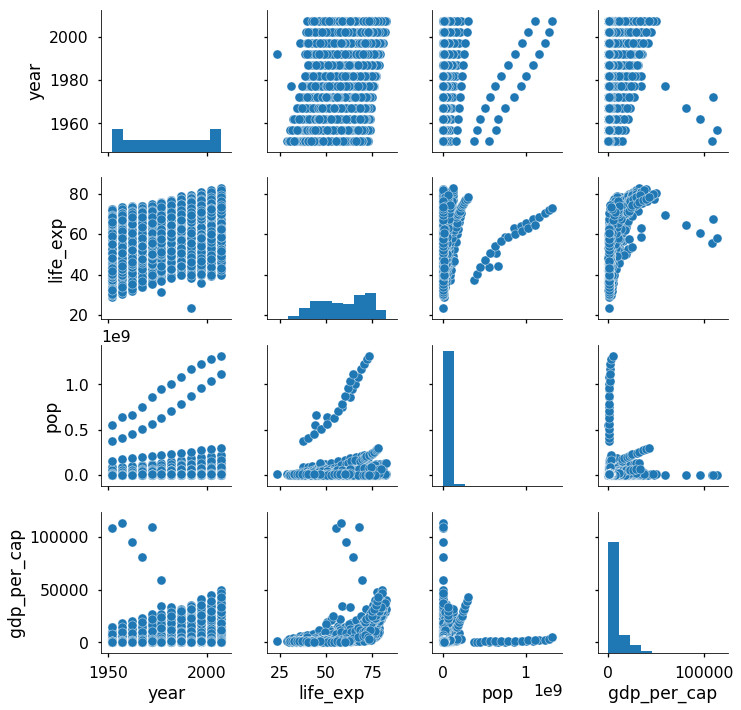# Take the log of population and gdp_per_capita
df['log_pop'] = np.log10(df['pop'])
df['log_gdp_per_cap'] = np.log10(df['gdp_per_cap'])

# Drop the non-transformed columns
df = df.drop(columns = ['pop', 'gdp_per_cap'])

sns.pairplot(df, hue = 'continent')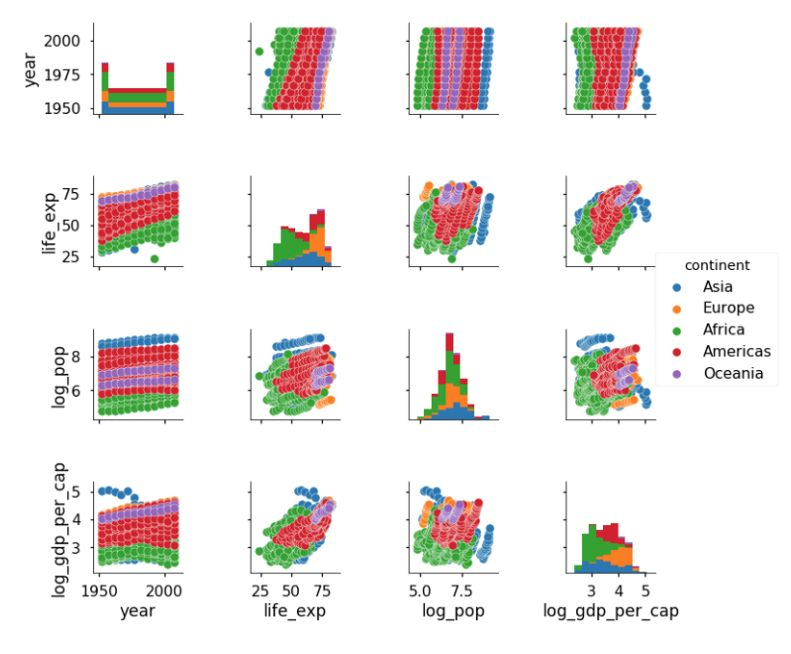# Create a pair plot colored by continent with a density plot of the # diagonal and format the scatter plots.
sns.pairplot(df, hue = 'continent', diag_kind = 'kde',
plot_kws = {'alpha': 0.6, 's': 80, 'edgecolor': 'k'},
size = 4)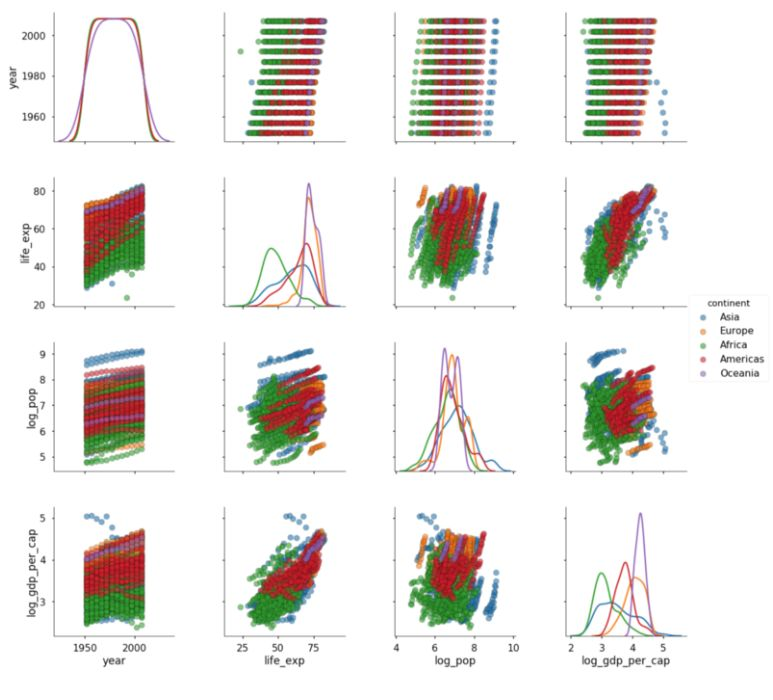# Plot colored by continent for years 2000-2007
sns.pairplot(df[df['year'] >= 2000],
vars = ['life_exp', 'log_pop', 'log_gdp_per_cap'],
hue = 'continent', diag_kind = 'kde',
plot_kws = {'alpha': 0.6, 's': 80, 'edgecolor': 'k'},
size = 4);
# Title
plt.suptitle('Pair Plot of Socioeconomic Data for 2000-2007',
size = 28);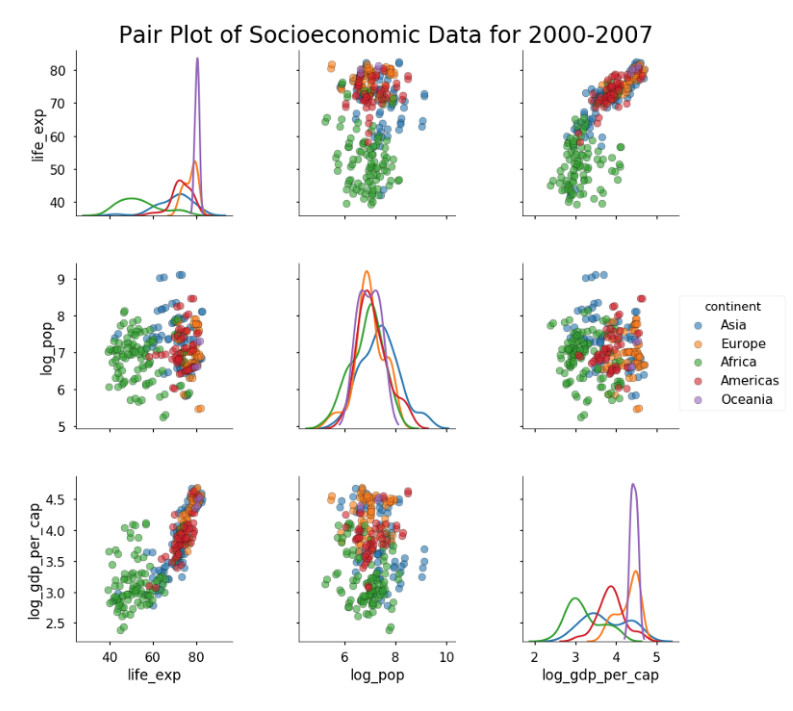# Create an instance of the PairGrid class.
grid = sns.PairGrid(data= df_log[df_log['year'] == 2007],
vars = ['life_exp', 'log_pop',
'log_gdp_per_cap'], size = 4)

# Map a scatter plot to the upper triangle
grid = grid.map_upper(plt.scatter, color = 'darkred')

map_upper 方法采用任意接受两个变量数组的函数（例如 plt.scatter），以及相关的关键词（例如 color）。map_lower 方法几乎与其相同，但是它填充的是网格的下三角。map_diag 与这两者稍有不同，因为它采用接受单个数组的函数（回想一下，对角线只显示单个变量）。一个例子是 plt.hist，我们使用它来填充对角线部分：

# Map a histogram to the diagonal
grid = grid.map_diag(plt.hist, bins = 10, color = 'darkred',
edgecolor = 'k')
# Map a density plot to the lower triangle
grid = grid.map_lower(sns.kdeplot, cmap = 'Reds')# Function to calculate correlation coefficient between two arrays
def corr(x, y, **kwargs):

# Calculate the value
coef = np.corrcoef(x, y)
# Make the label
label = r'$\rho$ = ' + str(round(coef, 2))

# Add the label to the plot
ax = plt.gca()
ax.annotate(label, xy = (0.2, 0.95), size = 20, xycoords = ax.transAxes)

# Create a pair grid instance
grid = sns.PairGrid(data= df[df['year'] == 2007],
vars = ['life_exp', 'log_pop', 'log_gdp_per_cap'], size = 4)

# Map the plots to the locations
grid = grid.map_upper(plt.scatter, color = 'darkred')
grid = grid.map_upper(corr)
grid = grid.map_lower(sns.kdeplot, cmap = 'Reds')
grid = grid.map_diag(plt.hist, bins = 10, edgecolor =  'k', color = 'darkred');